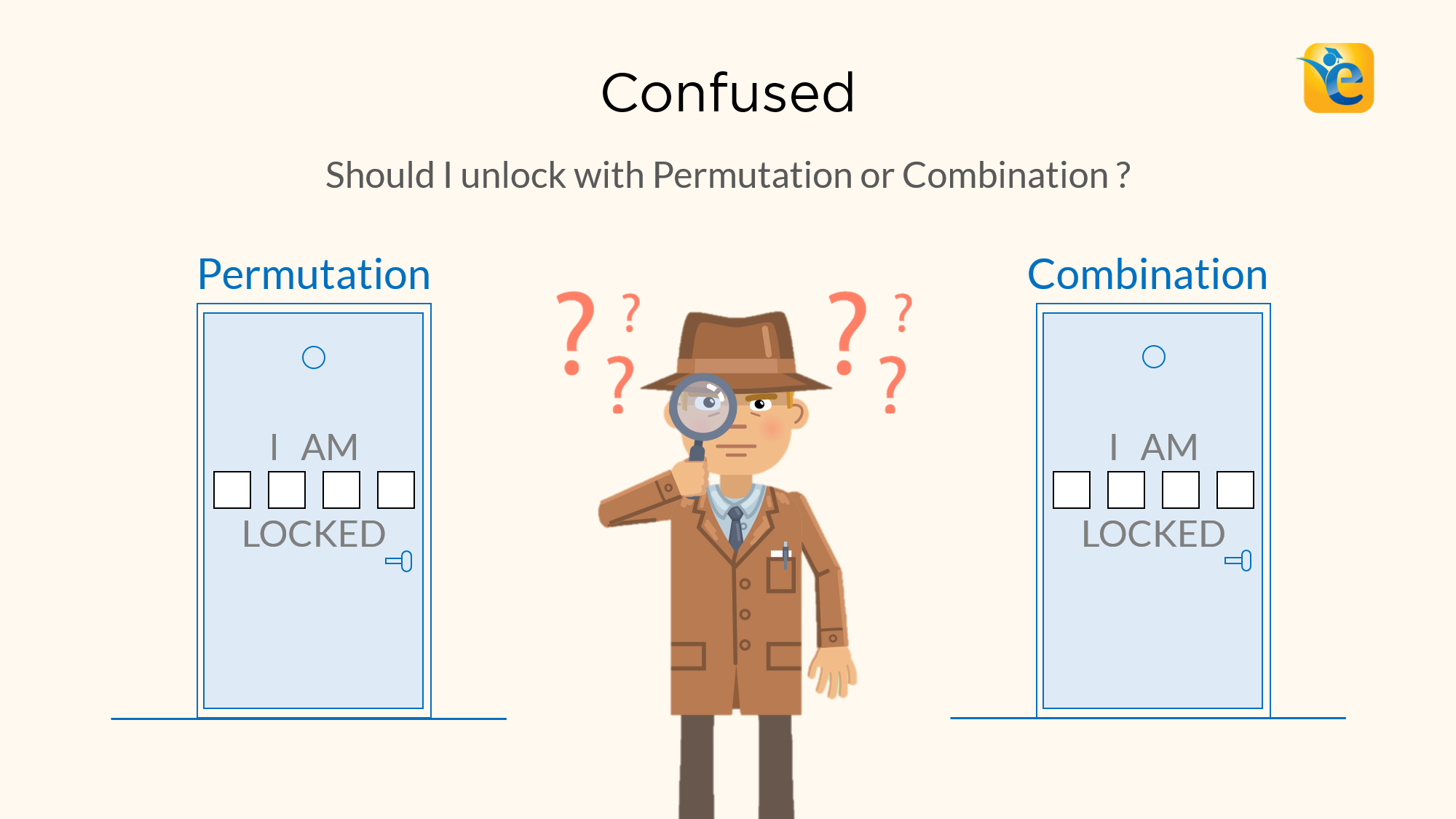# Difference between Permutation and Combination explained with examples

Permutations and Combinations is a topic full of conundrums. The biggest one is, understanding the difference between permutation and combination. Should I solve this question using permutation or combination? In this article, we will give you a foolproof method to differentiate between the two. In the last article of ‘Permutation and Combination’ series we talked

Read More# How to use Tree Structures to solve GMAT arithmetic problems

Read More# What was the population of City X in 2002? – OGQR 2020 Question #209 with Solution

[two_third] OGQR 2020: Question No. 209 What was the population of City X in 2002? X’s population in 2002 increased by 2 percent, or 20,000 people, over 2001. In 2001, X’s population was 1,000,000. [/two_third] [one_third_last] Source OGQR 2020 Type Data Sufficiency Topic Word Problems Sub-Topic Percentages Difficulty Medium [/one_third_last] Solution Steps 1 & 2: Understand Question

Read More# A certain painting job requires a mixture of yellow, green, and white paint. If 12 quarts of paint are needed for the job, how many quarts of green paint are needed? – OG 2020 Question #319 with Solution

[two_third] OG 2020: Question No. 319 A certain painting job requires a mixture of yellow, green, and white paint. If 12 quarts of paint are needed for the job, how many quarts of green paint are needed? The ratio of the amount of green paint to the amount of yellow and white paint combined needs

Read More# Jones has worked at Firm X twice as many years as Green, and Green has worked at Firm X four years longer than Smith. – OG 2020 Question #327 with Solution

[two_third] OG 2020: Question No. 327 Jones has worked at Firm X twice as many years as Green, and Green has worked at Firm X four years longer than Smith. How many years has Green worked at Firm X? Jones has worked at Firm X 9 years longer than Smith. Green has worked at Firm

Read More# A rectangular solid has length, width, and height of L cm, W cm, and H cm, respectively. If these dimensions are increased… – OG 2020 Question #376 with Solution

[two_third] OG 2020: Question No. 376 A rectangular solid has length, width, and height of L cm, W cm, and H cm, respectively. If these dimensions are increased by x%, y%, and z%, respectively, what is the percentage increase in the total surface area of the solid? L, W, and H are in the ratios

Read More# On a map, ½ inch represents 100 miles. According to this map, how many miles is City X from City Y? – OG 2020 Question #309 with Solution

[two_third] OG 2020: Question No. 309 On a map, ½ inch represents 100 miles. According to this map, how many miles is City X from City Y? City X is 3 inches from City Y on the map. Cities X and Y are each 300 miles from City Z. [/two_third] [one_third_last] Source OG 2020 Type

Read More# GMAT Word Problems | Application of Average Speed in Time – Distance Problems

GMAT Word problems on Time, and Speed, and Distance are based on the simple formula of  D = S x T, where D is the distance, S is speed and T is time. These GMAT word problems might look easy at first but if your concepts are not clear you will end up cringing in

Read More# GMAT Permutation and Combination | When to Add and Multiply

Read MoreRead More